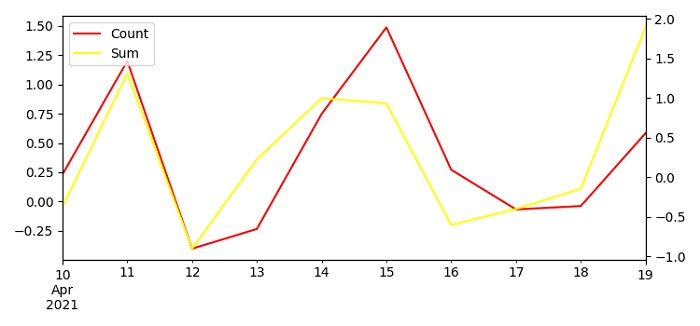# How to plot two Pandas time series on the same plot with legends and secondary Y-axis in Matplotlib?

To plot two Pandas time series on the sameplot with legends and secondary Y-axis, we can take the following steps −

• Set the figure size and adjust the padding between and around the subplots.

• Create a one-dimensional ndarray with axis labels (including time series).

• Make a dataframe with some column list.

• Plot columns A and B using dataframe plot() method.

• Return the handles and labels for the legend using get_legend_handles_labels() method.

• Place a legend on the figure using legend() method.

• To display the figure, use show() method.

## Example

import pandas as pd
from matplotlib import pyplot as plt
import numpy as np

plt.rcParams["figure.figsize"] = [7.50, 3.50]
plt.rcParams["figure.autolayout"] = True

ts = pd.Series(np.random.randn(10), index=pd.date_range('2021-04-10', periods=10))

df = pd.DataFrame(np.random.randn(10, 4), index=ts.index, columns=list('ABCD'))

ax1 = df.A.plot(color='red', label='Count')
ax2 = df.B.plot(color='yellow', secondary_y=True, label='Sum')

h1, l1 = ax1.get_legend_handles_labels()
h2, l2 = ax2.get_legend_handles_labels()

plt.legend(h1+h2, l1+l2, loc=2)

plt.show()

## Output